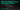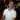# SUM with Multiple Criteria on the Same Column

October 04, 2017

While SUMIF and SUMIFS are great choices when working with conditional summing formulas, they fall short in one area. Specifically, they can’t be used to sum with multiple criteria on the same column. To be clear, this is specifically when you are trying to use the multiple criteria as an OR operation rather than an AND operation. When SUMIFS is used with multiple criteria, it’s like saying:

If column A has this AND this, then sum.

But if you need something like:

If column A has this OR this, then sum.

Then, well, you’re gonna have a bad time.## SUM with Multiple Criteria Using OR

Let’s say you had a simple table like the following:

(This is an Excel Table and it’s called Sales)

If you wanted to sum the sales based on an OR condition like “sum the sales if the region is East US OR West US,” how would you do that?

You may think to use SUMIFS() for this, but SUMIFS() apply multiple criteria with an AND operation:

``=SUMIFS(Sales[Sales (USD)],Sales[Region],"East US",Sales[Region],"West US")``

The problem here is that we’re trying to apply multiple criteria to the same column, which will be applied like an AND operation for SUMIFS().

The SUMIFS() function in this case says:

Sum the sales if the region is “East US” AND if the Region is “West US”

This could never be true so the equation evaluates to 0.

That’s not to say that SUMIFS() isn’t useful. You can apply multiple criteria to the same column so long as the logic makes sense. For example you could do this:

``=SUMIFS(Sales[Sales (USD)],Sales[Sales (USD)],">12000",Sales[Sales (USD)],"<15000")``

This would sum up the sales only if they were between \$12,000 and \$15,000.

So how can we apply multiple criteria to the same column with an OR logical condition?

### Use the plain old SUM() function

Poor SUM() function, it doesn’t get the popularity it deserves.

SUM() can do everything that SUMPRODUCT, SUMIF, SUMIFS, COUNT, COUNTIF, and COUNTIFS can do and more, but my guess is that people tend to find the syntax confusing and avoid trying to use it altogether.

I’m going to write another post in the future explaining how awesome the SUM() function really is, but for now we’ll keep the conversation on using it to replace SUMIFS().

To sum the sales if the region was East US OR West US, we can use the sum function like this:

``=SUM((Sales[Region]={"East US","West US"})*(Sales[Sales (USD)]))``

#### Breaking down the formula

There are two parts to this formula:

``=SUM((Sales[Region]={"East US","West US"})*(Sales[Sales (USD)]))``

In this section:

``(Sales[Region]={"East US","West US"})``

We have the multiple conditions that we will apply. And in this section:

``(Sales[Sales (USD)])``

We will match up the conditions with the corresponding sales numbers so we know which ones to sum up.

Let’s evaluate the conditions first:

``(Sales[Region]={"East US","West US"})``

The first thing that will happen is that the `Sales[Region]` will be shown as an array of values:

``({"East US","West US","West Europe"}={"East US","West US"})``

When we match up the values on the left with the values on the right, we get an array of boolean values:

``({TRUE,TRUE,FALSE})``

This is because “East US” on the left was found in the right (TRUE), the “West US” on the left was found in the right (TRUE), but “West Europe” was not found in the right side of the equation (FALSE).

Now let’s look at the `Sales[Sales (USD)]` side of the formula:

``=SUM(({TRUE,TRUE,FALSE})*(Sales[Sales (USD)]))``

This just ends up showing the values from the `Sales USD` column in the table.

``=SUM(({TRUE,TRUE,FALSE})*({14000,13000,15000}))``

Now all we have to do is sum up what’s TRUE:

``````=SUM(({TRUE,TRUE,FALSE})*({14000,13000,15000}))
=SUM(14000,13000)
=27000``````

And that’s how you can use SUM to apply multiple criteria on the same column.

Wow, you read the whole article! You know, people who make it this far are true learners. And clearly, you value learning. Would you like to learn more about Excel? Please consider supporting me by buying me a coffee (it takes a lot of coffee to write these articles!).Written by Joseph who loves teaching about Excel.